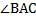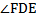### Sample Problem

Triangle ABC is an isosceles triangle,. Triangle DEF is a right triangle,. Findand.1)is °.

2)is °.

#### Solution

1) Since AB+ AC∠BAC=180° - ∠ABC -∠ACB=180° - 2∠ABC2)  Since DEF is a right triangle